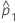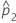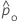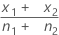# Methods and formulas for 2 Proportions

Select the method or formula of your choice.

## Confidence interval (CI)

### Notation

TermDescriptionestimate of the first population proportionestimate of the second population proportion
n1 number of trials in the first sample
n2 number of trials in the second sample
zα/2 inverse cumulative probability of the standard normal distribution at 1 – α/2
α 1 – confidence level/100

## Normal approximation test

The calculation of the test statistic, Z, depends on the method used to estimate p.

Separate estimates of p
By default, Minitab uses separate estimates of p for each population and calculates Z as follows:
Pooled estimate of p
If the hypothesized test difference is zero and you choose to use a pooled estimate of p for the test, Minitab calculates Z as follows:
The p-value for each alternative hypothesis is:
• H1: p1 > p2 : p-value = P(Z1z)
• H1: p1 < p2 : p-value = P(Z1 z)
• H1: p1p2 : p-value = 2P(Z1z)

Calculate these probabilities on the standard normal distribution.

### Notation

TermDescription
p1 true proportion of events in the first population
p2 true proportion of events in the second populationobserved proportion of events in the first sampleobserved proportion of events in the second sample
n1 number of trials in the first sample
n2 number of trials in the second sample
d0 hypothesized difference between the first and second proportionsx1 number of events in the first sample
x2 number of events in the second sample

## Fisher's exact test

Minitab performs Fisher's exact test in addition to a test based on a normal approximation. Fisher's exact test is valid for all sample sizes.

### Formula

Under the null hypothesis, the number of events in the first sample (x1) has a hypergeometric distribution with these parameters:
• Population size = n1 + n2
• Number of events in population = x1 + x2
• Sample size = n1
Let f( ) and F( ) denote the PDF and CDF of this hypergeometric distribution, respectively. Let Mode denote its mode. The p-values for each alternative hypothesis are as follows:
• H1: p1 < p2

p-value = F(x1)

• H1: p1 > p2

p-value = 1 – F(x1 – 1)

• H1: p1 p2
Three cases exist:
• Case 1: x1 < Mode
p-value = p-lower + p-upper
TermDescription
p-lower F(x1)
p-upper1 – F(y – 1)
y smallest integer > Mode such that f(y) <f(x1)
###### Note

p-upper may equal zero.

• Case 2: x1 = Mode

p-value = 1.0

• Case 3: x1 > Mode
p-value = p-lower + p-upper
TermDescription
p-upper1 – F(x1 – 1)
p-lower F(y)
y largest integer < Mode such that f(y) < f(x1)
###### Note

p-lower may equal zero.

### Notation

TermDescription
p1 true proportion of events in the first population
p2 true proportion of events in the second population
x1 number of events in the first sample
x2 number of events in the second sample
n1 number of trials in the first sample
n2 number of trials in the second sample
By using this site you agree to the use of cookies for analytics and personalized content.  Read our policy